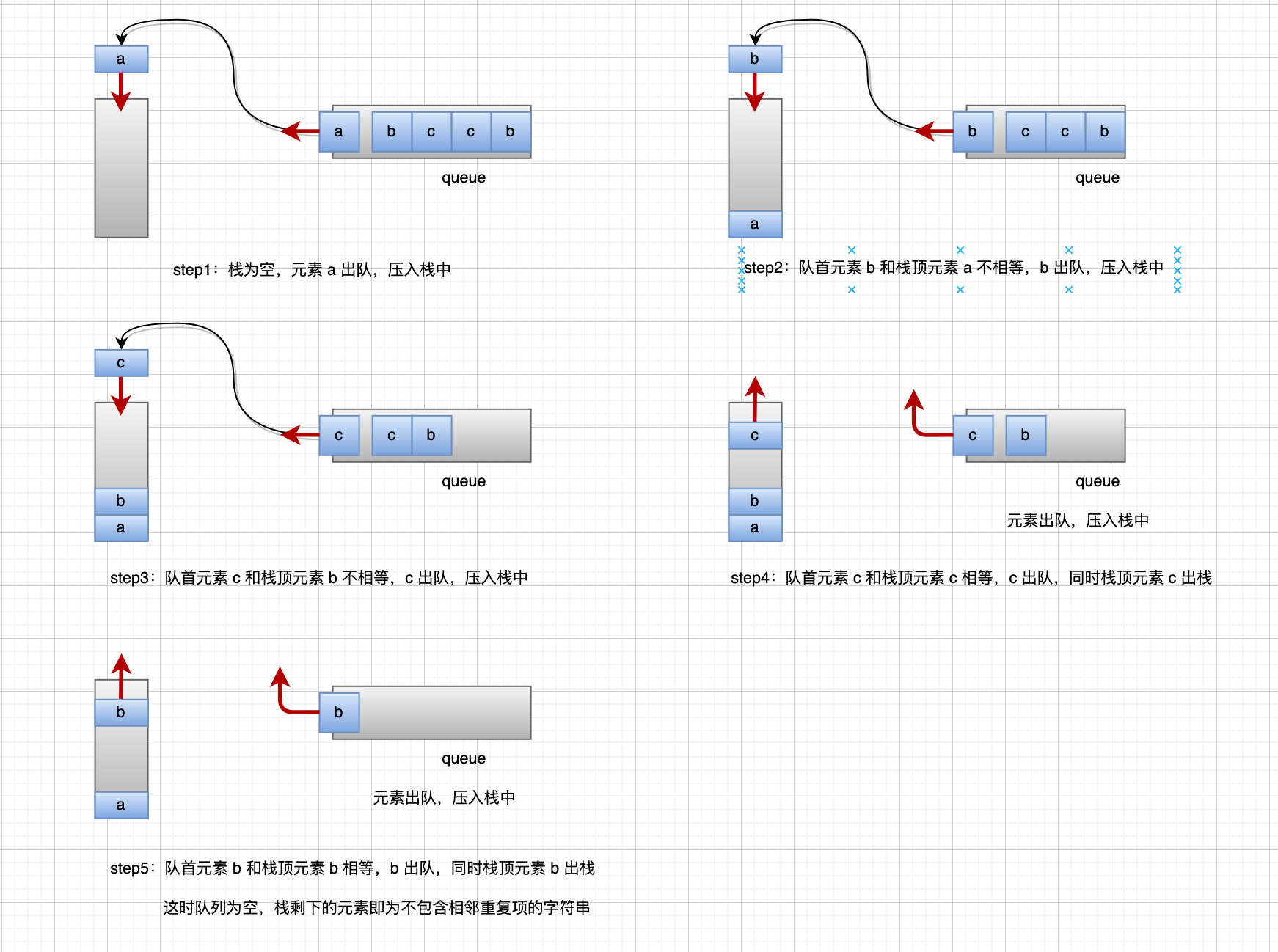# LeetCode#1047-Remove All Adjacent Duplicates In String-删除字符串中的所有相邻重复项

#### 一、题目

输入："abbaca"



1. 1 <= S.length <= 20000
2. S 仅由小写英文字母组成。

#### 二、题解

• 题解1：PHP自带的函数str_replace

function removeDuplicates($S) {$vowels = ['aa', 'bb', 'cc', 'dd', 'ee', 'ff', 'gg', 'hh', 'ii', 'jj', 'kk', 'll', 'mm', 'nn',
'oo', 'pp', 'qq', 'rr', 'ss', 'tt', 'uu', 'vv', 'ww', 'xx', 'yy', 'zz'];

while (strlen($S) >= 2) {$S = str_replace($vowels, "",$S);
if ($S == str_replace($vowels, "", $S)) { break; } } return$S;
}

• 题解2：出队入栈结合function removeDuplicates($S) { if (strlen($S) <= 1) {
return $S; }$stack = [];
$arr = str_split($S);
while (!empty($arr)) { if (empty($stack)) {
$stack[] = array_shift($arr);
} else {
if (current($arr) == end($stack)) {
array_shift($arr); array_pop($stack);
} else {
$stack[] = array_shift($arr);
}
}
}
return implode("", \$stack);
}

posted @ 2020-04-04 22:24  鹿呦呦  阅读(...)  评论(...编辑  收藏